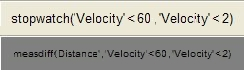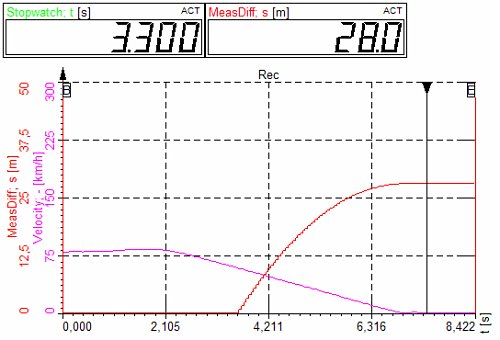# Example of stopwatch

There are two functions in Measure section of math which helps to measure time and value between events. The Stopwatch functions enable us to measure the time between a start and stop event. The ‘measdiff’ function makes the same measurement, but we can define the measurement channel instead of time.

In the example shown we measure the brake time and brake distance. The first example gives the time of braking between 60 km/h and 2 km/h.

The second channel measures the distance between 60 and 2 km/h. This is achieved by defining the Distance channel as the calculated value.The graph below shows the example of measured data - input channels of velocity and distance. The meter shows the time and distance of braking.Dewesoft 7 interpolates the velocity and distance channels. So for examples if measured velocity points are 61.2 and 59.2, it will take the time in between those two values as the start trigger.